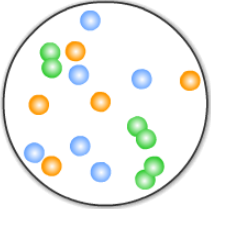# Problem: Consider this molecular-level representation of a gas.If the partial pressure of the diatomic gas is 0.480 atm, what is the total pressure?

###### FREE Expert Solution

To calculate total pressure Ptot

Recall that the partial pressure of a gas (PGas) in a mixture is given by:

$\overline{){{\mathbf{P}}}_{{\mathbf{gas}}}{\mathbf{=}}{{\mathbf{\chi }}}_{{\mathbf{gas}}}{{\mathbf{P}}}_{{\mathbf{total}}}}$

where χGas = mole fraction of the gas and Ptotal = total pressure of the gas mixture

The mole fraction of the gas is then given by:###### Problem Details

Consider this molecular-level representation of a gas.If the partial pressure of the diatomic gas is 0.480 atm, what is the total pressure?